# waynehu

## Professor, Department of Astronomy and Astrophysics University of Chicago

Group Contact CV SnapShots

So far we have been neglecting the baryons in the dynamics of the acoustic oscillations. To see whether this is a reasonable approximation consider the photon-baryon momentum density ratio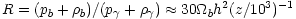. For typical values of the baryon density this number is of order unity at recombination and so we expect baryonic effects to begin appearing in the oscillations just as they are frozen in.

Baryons are conceptually easy to include in the evolution equations since their momentum density provides extra inertia in the joint Euler equation for pressure and potential gradients to overcome. Since inertial and gravitational mass are equal, all terms in the Euler equation save the pressure gradient are multiplied by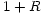leading to the revised oscillator equation [Hu & Sugiyama, 1995]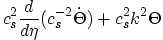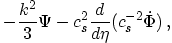(16)

where we have used the fact that the sound speed is reduced by the baryons to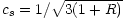.

To get a feel for the implications of the baryons take the limit of constant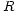,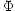and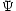. Then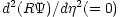may be added to the left hand side to again put the oscillator equation in the form of Equation (9) with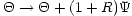. The solution then becomes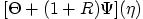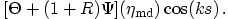(17)

Aside from the lowering of the sound speed which decreases the sound horizon, baryons have two distinguishing effects: they enhance the amplitude of the oscillations and shift the equilibrium point to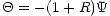(see Figure 1b). These two effects are intimately related and are easy to understand since the equations are exactly those of a mass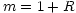on a spring in a constant gravitational field. For the same initial conditions, increasing the mass causes the oscillator to fall further in the gravitational field leading to larger oscillations and a shifted zero point.

The shifting of the zero point of the oscillator has significant phenomenological consequences. Since it is still the effective temperature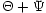that is the observed temperature, the zero point shift breaks the symmetry of the oscillations. The baryons enhance only the compressional phase, i.e. every other peak. For the working cosmological model these are the first, third, fifth... Physically, the extra gravity provided by the baryons enhance compression into potential wells.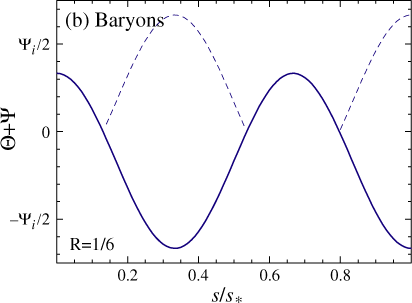Figure: Idealized acoustic oscillations. (b) Baryon loading. Baryon loading boosts the amplitudes of every other oscillation. Plotted here is the idealization of Equation (16) (constant potentials and baryon loading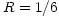) for the third peak.

These qualitative results remain true in the presence of a time-variable. An additional effect arises due to the adiabatic damping of an oscillator with a time-variable mass. Since the energy/frequency of an oscillator is an adiabatic invariant, the amplitude must decay as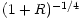. This can also be understood by expanding the time derivatives in Equation (16) and identifying the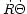term as the remnant of the familiar expansion drag on baryon velocities.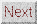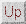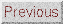Next: Radiation Driving Up: ACOUSTIC PEAKS Previous: Gravitational Forcing
Wayne Hu 2001-10-15